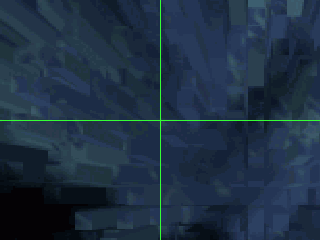FİZİKA PROBLEMLƏRİ İNSTİTUTU## HIGH ENERGY PHYSICS

Investigations mainly cover theoretical and phenomenological problems of Quantum Chromodynamics. In order to describe strong interactions of hadrons both the perturbative and nonperturbative QCD methods are employed. The Nambu-Jona-Lasinio model is also used for modeling of hadronic states. Problems of the Yang-Mills quantum mechanics are considered in the numerous external backgrounds. Ideas of the AdS/CFT correspondence are started to use for solution of various problems in the quantum field theory.

TOPICS OF INVESTIGATION

• Perturbative QCD: exclusive and inclusive processes
• Holographic QCD
• Theory of the Yang-Mills fields
• Chiral models
• Mathematical methods in elementary particle physics

MAIN RESULTS

The method for estimation of end-point power corrections in exclusive processes- the running coupling method- was developed. This method allowed us to compute such corrections to light mesons electromagnetic and transition form factors, and other processes.

The pion twist-4 distribution amplitudes were found. The obtained distribution amplitudes were employed within the QCD light-cone sum rule method for calculation of higher twist corrections to the pion form factors. Comparison of theoretical predictions with available experimental data was done. Details can be found in my works.

Method for solution of the Bethe-Salpeter ladder integral equations for the scattering amplitude in some scalar and spinor models was constructed and universal solution of these equations in the Regge asymptotical form is found.

Two variants of the Nambu-Jona-Lasinio model - the model with 4-dimensional cutoff and the model with dimensional regularization were systematically compared. It was shown that they are, in general, two different models of light-quark interaction. In the mean-field approximation the distinction between them becomes apparent in the behavior of the scalar amplitude near the threshold. For 4-dimensional cutoff the pole term can be extracted, which corresponds to sigma-meson. For dimensional regularization the singularity of the scalar amplitude is not a pole, but it disappears at some value of the regularization parameter. Still more essential distinction of these models exists in the next-to-leading order (NLO) in the mean-field expansion. Calculations of meson contributions in the quark chiral condensate and in the dynamical quark mass demonstrate, that these contributions though their relatively smallness can destabilize the Nambu-Jona-Lasinio model with 4-dimensional cutoff. On the contrary, the Nambu-Jona-Lasinio model with dimensional regularization is stabilized in NLO, i.e., the value of the regularization parameter shifts to the stability region, where these contributions are defined and the NLO pion correction to quark mass in both regularizations is equal to zero.

Motion of a colored Dirac particle was considered in a constant chromomagnetic field. Dirac equation for such particle was solved and spectra of the particle moving inside of the cylinder or sphere backgrounds were found. In the axial field motion takes place on circular orbits and in spherical field it takes place on well- known s p d f … orbitals. By unitary transformation the squared Dirac equation was diagonalized and wave function of states with definite energy was found. For the case of the diagonal Hamiltonian the supersymmetry of the Dirac equation and superpartnerships of the energy states were established. A configuration of background chromomagnetic and chromoelectric fields were used for construction of a toy bag model of hadrons.

AdS/CFT correspondence of R-current correlators was studied using the world-line formalism. Two and three point correlators of the vector field were matched with the corresponding correlators of AdS supergravity. Contributions of scalar and spinor loops were taken into account and expressed in terms of bulk to boundary propagators.

The group properties of the equations of relativistic and non relativistic quantum oscillators were investigated by Lie group methods and their invariant solutions were found. The operator which translates quantum solutions to classical ones was constructed.

The problem of group classification for nonlinear classical oscillator in arbitrary potential was solved. This nonlinear equation was transformed to linear equations, and exact solutions of the latter were obtained. New symmetry group of the Born-Infeld equation was found and its new invariant solutions were computed. Action of symmetry groups on the conservation laws was investigated and new algorithm for construction of the basis for conservation laws was proposed.

A model for magneto-acoustic-gravity waves was proposed, its group properties were investigated. Effects of magnetic and gravitational fields on symmetry groups were studied. It was demonstrated, that inclusion of a magnetic field into equations does not change their symmetry group, whereas inclusion of the gravity field leads to appearance of additional symmetry and, as a results to changes in the symmetry groups.